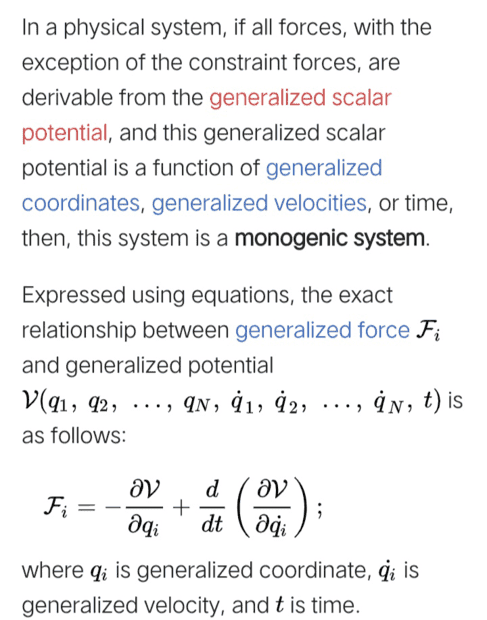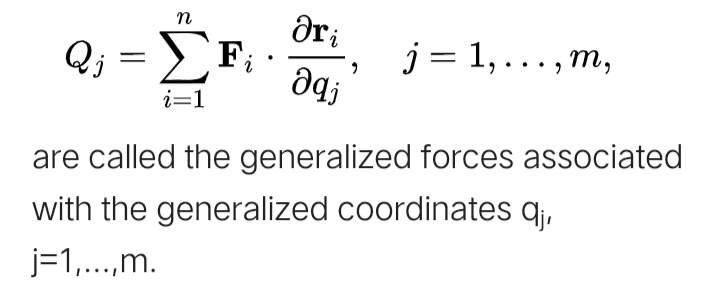# Two equations of generalized forces

• A
Kashmir
Wikipedia article under generalized forces saysAlso we know that the generalized forces are defined asHow can I derive the first equation from the second for a monogenic system ?

Homework Helper
What is the definition of Fi in the second equation?

Kashmir
What is the definition of Fi in the second equation?
##F_i## is the force on the ith particle

ergospherical
You have it the wrong way around! There are some mechanical systems for which ##Q_j## can be written in the form ##Q_j = \dfrac{d}{dt} \dfrac{\partial U}{\partial \dot{q}^j} - \dfrac{\partial U}{\partial q^j}##, where ##U## is called a velocity dependent potential.

[for example, the Lorentz force is derivable from the velocity dependent potential ##U = q\phi - q \dot{\mathbf{r}}\cdot \mathbf{A}##]

If this is the case, then it follows that Lagrange's equation ## \dfrac{d}{dt} \dfrac{\partial T}{\partial \dot{q}^j} - \dfrac{\partial T}{\partial q^j} = Q_j## can be written in Lagrangian form by taking ##L(q,\dot{q},t) = T(q,\dot{q},t) - U(q,\dot{q},t)##.

•vanhees71
Kashmir
You have it the wrong way around! There are some mechanical systems for which ##Q_j## can be written in the form ##Q_j = \dfrac{d}{dt} \dfrac{\partial U}{\partial \dot{q}^j} - \dfrac{\partial U}{\partial q^j}##, where ##U## is called a velocity dependent potential.

[for example, the Lorentz force is derivable from the velocity dependent potential ##U = q\phi - q \dot{\mathbf{r}}\cdot \mathbf{A}##]

If this is the case, then it follows that Lagrange's equation ## \dfrac{d}{dt} \dfrac{\partial T}{\partial \dot{q}^j} - \dfrac{\partial T}{\partial q^j} = Q_j## can be written in Lagrangian form by taking ##L(q,\dot{q},t) = T(q,\dot{q},t) - U(q,\dot{q},t)##.
You have it the wrong way around! There are some mechanical systems for which ##Q_j## can be written in the form ##Q_j = \dfrac{d}{dt} \dfrac{\partial U}{\partial \dot{q}^j} - \dfrac{\partial U}{\partial q^j}##, where ##U## is called a velocity dependent potential.

[for example, the Lorentz force is derivable from the velocity dependent potential ##U = q\phi - q \dot{\mathbf{r}}\cdot \mathbf{A}##]

If this is the case, then it follows that Lagrange's equation ## \dfrac{d}{dt} \dfrac{\partial T}{\partial \dot{q}^j} - \dfrac{\partial T}{\partial q^j} = Q_j## can be written in Lagrangian form by taking ##L(q,\dot{q},t) = T(q,\dot{q},t) - U(q,\dot{q},t)##.
Thank you for your reply but Why have I it the wrong way around ?
You also seem to say the same thing which I'm saying.

In all cases isn't the generalized force ##Q_{j}=\sum_{i=1}^{n} \mathbf{F}_{i} \cdot \frac{\partial \mathbf{r}_{i}}{\partial q_{j}}## and for some situations (monogenic) it reduces to ##\mathcal{F}_{i}=-\frac{\partial \mathcal{V}}{\partial q_{i}}+\frac{d}{d t}\left(\frac{\partial \mathcal{V}}{\partial \dot{q}_{i}}\right)## which I can't prove how.
Please tell me what I'm doing wrong. I don't have a teacher.
God bless you

ergospherical
that’s correct. in some (special) cases the generalised force takes that second form. there’s nothing to prove!

•Kashmir
Kashmir
that’s correct. in some (special) cases the generalised force takes that second form. there’s nothing to prove!
Maybe I'm not clear.
Do you agree that we have defined generalized forces In all cases as ##Q_{j}=\sum_{i=1}^{n} \mathbf{F}_{i} \cdot \frac{\partial \mathbf{r}_{i}}{\partial q_{j}}## .

If that's true then the definition of generalized forces for monogenic systems is the same.
But it's said that the generalized force in the monogenic system is different given as ##\mathcal{F}_{i}=-\frac{\partial \mathcal{V}}{\partial q_{i}}+\frac{d}{d t}\left(\frac{\partial \mathcal{V}}{\partial \dot{q}_{i}}\right)##.

So there is a contradiction. And the only way to resolve it is to prove that they are equivalent for the monogenic case,which I cant.

I hope I'm clear. Thank you again :)

Kashmir
that’s correct. in some (special) cases the generalised force takes that second form. there’s nothing to prove!
What are those special cases? Under those special cases we should be able to move from ##Q_{j}=\sum_{i=1}^{n} \mathbf{F}_{i} \cdot \frac{\partial \mathbf{r}_{i}}{\partial q_{j}}## to ##\mathcal{F}_{i}=-\frac{\partial \mathcal{V}}{\partial q_{i}}+\frac{d}{d t}\left(\frac{\partial \mathcal{V}}{\partial \dot{q}_{i}}\right)##

ergospherical
e.g. the Lorentz force
for example take the generalised coordinates to be the cartesian coordinates ##\mathbf{r} = (x,y,z)##
and let ##U(\mathbf{r}, \dot{\mathbf{r}}) = q\phi(\mathbf{r}) - q\dot{\mathbf{r}} \cdot \mathbf{A}(\mathbf{r})## be a velocity dependent potential

then ##\dfrac{\partial U}{\partial x_j} = q\dfrac{\partial \phi}{\partial x_j} - q\dot{x}_i \dfrac{\partial A_i}{\partial x_j} ##
and ##\dfrac{d}{dt} \dfrac{\partial U}{\partial \dot{x}_j} = -q \dfrac{dA_j}{dt}##
therefore\begin{align*}
Q_j = \dfrac{d}{dt} \dfrac{\partial U}{\partial \dot{x}_j} - \dfrac{\partial U}{\partial x_j} &= -q \dfrac{dA_j}{dt} - q\dfrac{\partial \phi}{\partial x_j} + q\dot{x}_i \dfrac{\partial A_i}{\partial x_j} \\

&= q \dot{x}_i \left( \dfrac{\partial A_i}{\partial x_j} - \dfrac{\partial A_j}{\partial x_i}\right) - q\dfrac{\partial \phi}{\partial x_j}
\end{align*}the first term can be rewritten as\begin{align*}
[\dot{\mathbf{r}} \times (\nabla \times \mathbf{A})]_j &= \epsilon_{jkl} \dot{x}_k (\nabla \times \mathbf{A})_l \\
&= \epsilon_{jkl} \dot{x}_k \epsilon_{lmn} \dfrac{\partial A_n}{\partial x_m} \\
&= (\delta_{jm} \delta_{kn} - \delta_{km} \delta_{jn})\dot{x}_k \dfrac{\partial A_n}{\partial x_m} \\
&= \dot{x}_k \left( \dfrac{\partial A_k}{\partial x_j} -\dfrac{\partial A_j}{\partial x_k} \right)
\end{align*}(just replace the dummy index ##k \rightarrow i##), and putting ##\nabla \times \mathbf{A} \equiv \mathbf{B}## as well as ##-\dfrac{\partial \phi}{\partial \mathbf{r}} \equiv \mathbf{E}## gives\begin{align*}
Q_j = q (\dot{\mathbf{r}} \times \mathbf{B})_j + qE_j
\end{align*}i.e. the Lorentz force

it's easy for this example, in the case of cartesian coordinates, to see that the generalised force is nothing but the actual force ##Q_j = \mathbf{F} \cdot \dfrac{\partial \mathbf{r}}{\partial x_j} = \mathbf{F} \cdot \mathbf{e}_j = F_j##.

•Kashmir, dextercioby and vanhees71
$$Q_i=(\boldsymbol F,\frac{\partial \boldsymbol v_A}{\partial \dot q_i})+(\boldsymbol M_A,\frac{\partial \boldsymbol \omega}{\partial \dot q_i})$$